Call Back24x7 Support Available

chat now

In A Hurry? Get A Callback

# Creating a Minitab DatabaseAssignment #2: Descriptive Statistics

Student Name

College Name

Summary

Descriptive Statistics can be used for couple of reasons. One is to provide basic statistical information about the dataset and the other is to highlight significant relationships between the variables from the dataset. Basic statistics helps us understanding the behavior of the data. When we say descriptive statistics, it comprises of two types of measures – One is central tendency measures such as mean, median and mode and the other is dispersion or variation measures such as variance, standard deviation, standard error, etc.

The given data set from Week 1 project comprises of id number, age, sex, height and year in college. Amongst these variables, id number is alphanumeric as it is the unique of the dataset. Age and height are the two continuous variables and hence are numeric in nature whereas sex and year in college are categorical variables and are nominal in nature. There are 40 observations in the total dataset.

Using Minitab, we have computed the basic descriptive statistics both numerical and graphical representation to describe the variables under consideration.  From the below summary, we can say that the average of age is 19.5 years with 1.132 deviation between each observation. On the other hand, the average height is 65.025 inches with 4.649 deviation between each observation. The minimum height is 53 inches and the maximum height is 73 inches respectively. When it comes to skewness, we can say the age symmetrical and has normal distribution due to zero skewness. Whereas it case of height, it is negatively skewed and has long left tail as depicted by the below visual representations.

### Live web tutors

A reputable assignment writing service.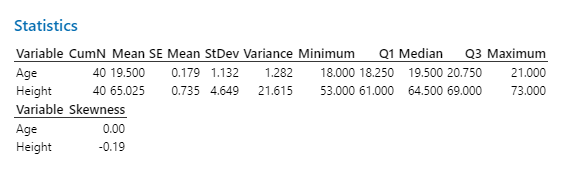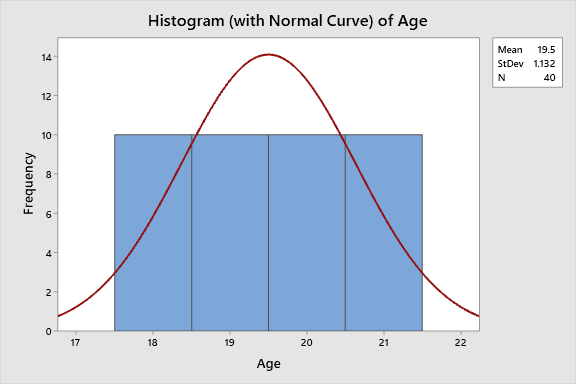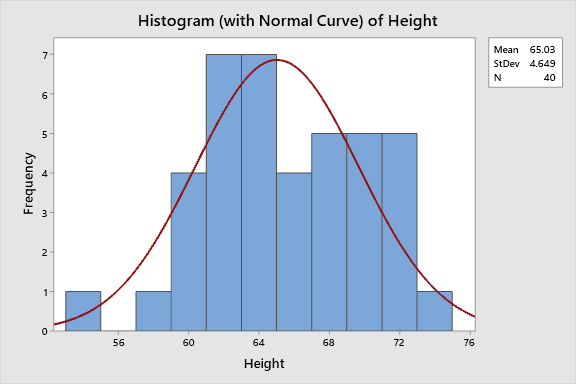We have also carried out analysis of height by each categorical variable. First, we will study the height of male and female individually.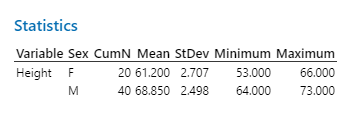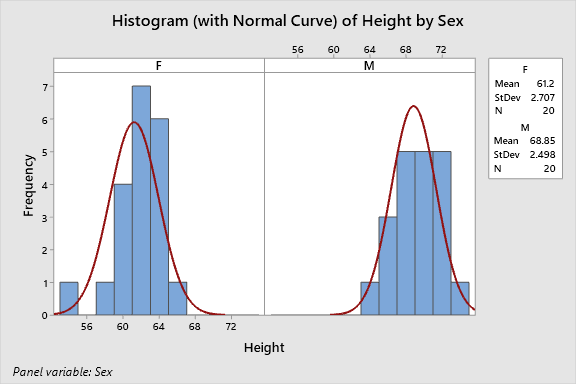By looking at the numbers, we can say that the average height is 61.2 inches for females with maxium at 66 inches and minimum at 53 inches. Whereas on the other hand, the average height of male students is 68.85 inches with maximum at 73 inches and minium at 64 inches respectively.

Next, we are doing height by year in college namely, freshman, sophomore, junior and senior. From the below table, we can see that the tallest student is in Freshman section and student is small height is in Sophomore section.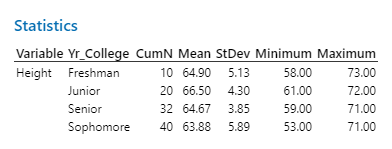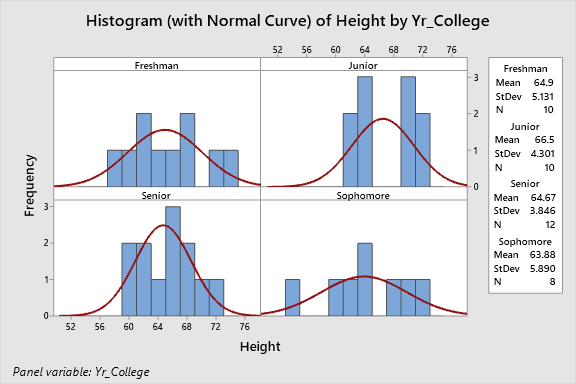References

• Gina Sigler (2018), Statistics Reference Series Part 1: Descriptive Statistics
• Ali Z, Bhaskar SB. Basic statistical tools in research and data analysis. Indian J Anaesth 2016;60:662-9.

Assignment #3: Descriptive Statistics

Student Name

College Name

Summary

Descriptive Statistics can be used for couple of reasons. One is to provide basic statistical information about the dataset and the other is to highlight significant relationships between the variables from the dataset. Basic statistics helps us understanding the behavior of the data. When we say descriptive statistics, it comprises of two types of measures – One is central tendency measures such as mean, median and mode and the other is dispersion or variation measures such as variance, standard deviation, standard error, etc.

The given data set from Week 1 project comprises of id number, age, sex, height and year in college. Amongst these variables, id number is alphanumeric as it is the unique of the dataset. Age and height are the two continuous variables and hence are numeric in nature whereas sex and year in college are categorical variables and are nominal in nature. But now, instead of height and year in college, we are using ethnicity, whether student is African or American. There are 40 observations in the total dataset.

Using Minitab, we have computed the basic descriptive statistics both numerical and graphical representation to describe the variables under consideration. First we will do the analysis for the age and ethnicity for Female students.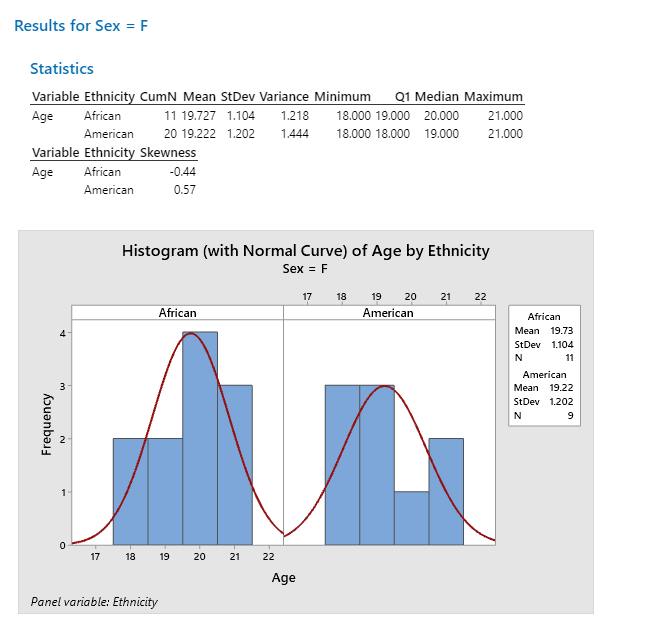By looking at the numerical statistical summary, we can say that the average age African student is 19.727 years whereas for Americans, it is 19.222 years. The skewness of age for African is negative and for Americans it is positive. This means that most of the African students lies to the left of the average age whereas Americans are towards the right of average age and hence is skewed to the right in the normal distribution curve with histogram as shown below.

### We Can Help!

View Some Assignment Samples

Now, we will do the same for Male students with ethnicity under consideration.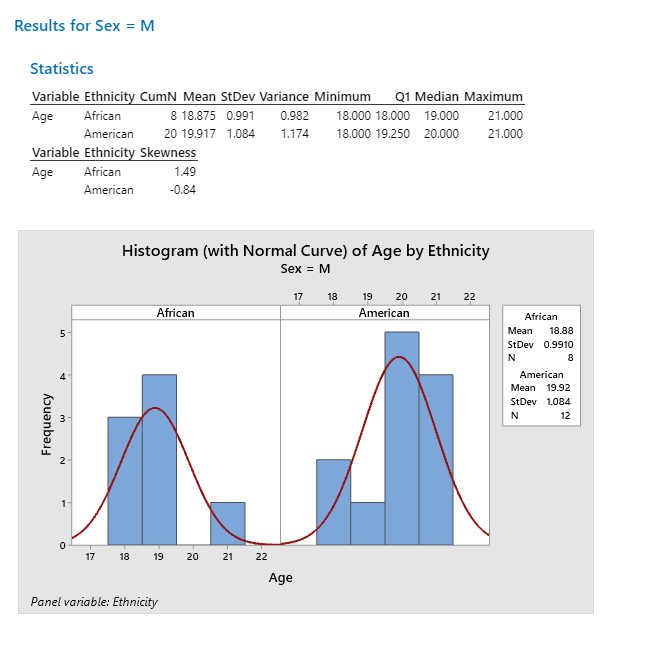Similar to female analysis, here also we can see that the average age African student is 18.875 years whereas for Americans, it is 19.917 years. The skewness of age for African is positive for males and for Americans it is negative as opposite to what we have seen in females. This means that most of the African students’ age lies to the right of the average age whereas Americans are towards the left of average age and hence normal curves are shown below.

We have also created the table for categorical variables, namely, age and ethnicity. We can see that African females are 27.5% and American are 22.5% amongst total students and African males are 47.5% and American males are 52.5%.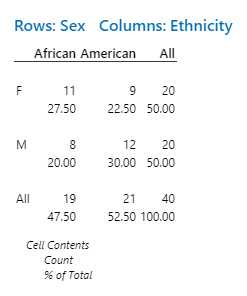References

• Gina Sigler (2018), Statistics Reference Series Part 1: Descriptive Statistics
• Ali Z, Bhaskar SB. Basic statistical tools in research and data analysis. Indian J Anaesth 2016;60:662-9.